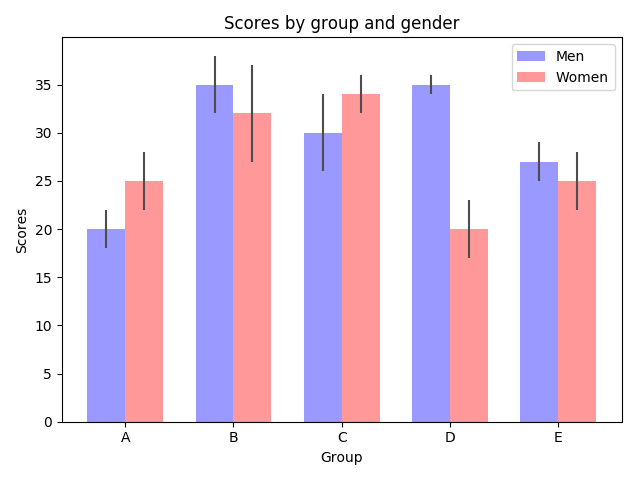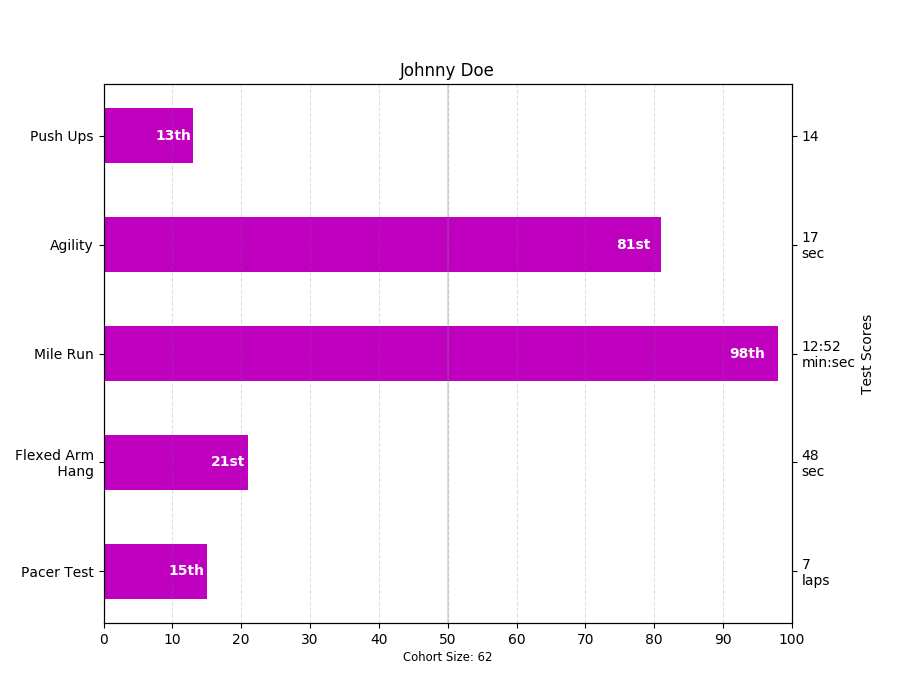Travis-CI:# Barchart Demo¶

Bar charts of many shapes and sizes with Matplotlib.

Bar charts are useful for visualizing counts, or summary statistics with error bars. These examples show a few ways to do this with Matplotlib.

```# Credit: Josh Hemann

import numpy as np
import matplotlib.pyplot as plt
from matplotlib.ticker import MaxNLocator
from collections import namedtuple

n_groups = 5

means_men = (20, 35, 30, 35, 27)
std_men = (2, 3, 4, 1, 2)

means_women = (25, 32, 34, 20, 25)
std_women = (3, 5, 2, 3, 3)

fig, ax = plt.subplots()

index = np.arange(n_groups)
bar_width = 0.35

opacity = 0.4
error_config = {'ecolor': '0.3'}

rects1 = ax.bar(index, means_men, bar_width,
alpha=opacity, color='b',
yerr=std_men, error_kw=error_config,
label='Men')

rects2 = ax.bar(index + bar_width, means_women, bar_width,
alpha=opacity, color='r',
yerr=std_women, error_kw=error_config,
label='Women')

ax.set_xlabel('Group')
ax.set_ylabel('Scores')
ax.set_title('Scores by group and gender')
ax.set_xticks(index + bar_width / 2)
ax.set_xticklabels(('A', 'B', 'C', 'D', 'E'))
ax.legend()

fig.tight_layout()
plt.show()
```This example comes from an application in which grade school gym teachers wanted to be able to show parents how their child did across a handful of fitness tests, and importantly, relative to how other children did. To extract the plotting code for demo purposes, we’ll just make up some data for little Johnny Doe…

```Student = namedtuple('Student', ['name', 'grade', 'gender'])
Score = namedtuple('Score', ['score', 'percentile'])

# GLOBAL CONSTANTS
testNames = ['Pacer Test', 'Flexed Arm\n Hang', 'Mile Run', 'Agility',
'Push Ups']
testMeta = dict(zip(testNames, ['laps', 'sec', 'min:sec', 'sec', '']))

def attach_ordinal(num):
"""helper function to add ordinal string to integers

1 -> 1st
56 -> 56th
"""
suffixes = dict((str(i), v) for i, v in
enumerate(['th', 'st', 'nd', 'rd', 'th',
'th', 'th', 'th', 'th', 'th']))

v = str(num)
# special case early teens
if v in {'11', '12', '13'}:
return v + 'th'
return v + suffixes[v[-1]]

def format_score(scr, test):
"""
Build up the score labels for the right Y-axis by first
the appropriate meta information (i.e., 'laps' vs 'seconds'). We
want the labels centered on the ticks, so if there is no meta
the string
"""
md = testMeta[test]
if md:
return '{0}\n{1}'.format(scr, md)
else:
return scr

def format_ycursor(y):
y = int(y)
if y < 0 or y >= len(testNames):
return ''
else:
return testNames[y]

def plot_student_results(student, scores, cohort_size):
#  create the figure
fig, ax1 = plt.subplots(figsize=(9, 7))

pos = np.arange(len(testNames))

rects = ax1.barh(pos, [scores[k].percentile for k in testNames],
align='center',
height=0.5, color='m',
tick_label=testNames)

ax1.set_title(student.name)

ax1.set_xlim([0, 100])
ax1.xaxis.set_major_locator(MaxNLocator(11))
ax1.xaxis.grid(True, linestyle='--', which='major',
color='grey', alpha=.25)

# Plot a solid vertical gridline to highlight the median position
ax1.axvline(50, color='grey', alpha=0.25)
# set X-axis tick marks at the deciles
cohort_label = ax1.text(.5, -.07, 'Cohort Size: {0}'.format(cohort_size),
horizontalalignment='center', size='small',
transform=ax1.transAxes)

# Set the right-hand Y-axis ticks and labels
ax2 = ax1.twinx()

scoreLabels = [format_score(scores[k].score, k) for k in testNames]

# set the tick locations
ax2.set_yticks(pos)
# make sure that the limits are set equally on both yaxis so the
# ticks line up
ax2.set_ylim(ax1.get_ylim())

# set the tick labels
ax2.set_yticklabels(scoreLabels)

ax2.set_ylabel('Test Scores')

ax2.set_xlabel(('Percentile Ranking Across '
gender=student.gender.title()))

rect_labels = []
# Lastly, write in the ranking inside each bar to aid in interpretation
for rect in rects:
# Rectangle widths are already integer-valued but are floating
# type, so it helps to remove the trailing decimal point and 0 by
# converting width to int type
width = int(rect.get_width())

rankStr = attach_ordinal(width)
# The bars aren't wide enough to print the ranking inside
if (width < 5):
# Shift the text to the right side of the right edge
xloc = width + 1
# Black against white background
clr = 'black'
align = 'left'
else:
# Shift the text to the left side of the right edge
xloc = 0.98*width
# White on magenta
clr = 'white'
align = 'right'

# Center the text vertically in the bar
yloc = rect.get_y() + rect.get_height()/2.0
label = ax1.text(xloc, yloc, rankStr, horizontalalignment=align,
verticalalignment='center', color=clr, weight='bold',
clip_on=True)
rect_labels.append(label)

# make the interactive mouse over give the bar title
ax2.fmt_ydata = format_ycursor
# return all of the artists created
return {'fig': fig,
'ax': ax1,
'ax_right': ax2,
'bars': rects,
'perc_labels': rect_labels,
'cohort_label': cohort_label}

student = Student('Johnny Doe', 2, 'boy')
scores = dict(zip(testNames,
(Score(v, p) for v, p in
zip(['7', '48', '12:52', '17', '14'],
np.round(np.random.uniform(0, 1,
len(testNames))*100, 0)))))
cohort_size = 62  # The number of other 2nd grade boys

arts = plot_student_results(student, scores, cohort_size)
plt.show()
```Total running time of the script: ( 0 minutes 0.083 seconds)

Gallery generated by Sphinx-Gallery